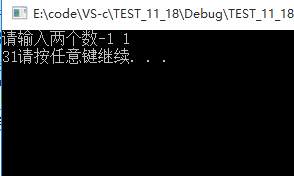# 求二进制序列中1的个数及奇偶序列+输出整数的每一位+两个数中多少个bit位不同

2017-11-18 15:37 25 查看

```int main()
{
int count = 0;
int num = 0;
printf("输入一个数:");
scanf("%d", &num);
while (num)
{
if (num % 2 == 1)
{
count++;
}
num = num / 2;
}
printf("1的个数为%d", count);
system("pause");
return 0;
}
//可以测试正数，负数无法测试```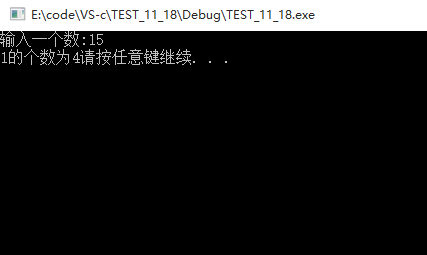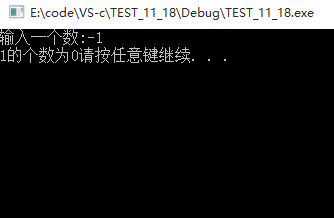```int main()
{
int i = 0;
int num = 0, count = 0;
printf("请输入一个数：");
scanf("%d", &num);
for (i = 0; i < 32; i++)
{
if ((num >> i) & 1 == 1)
{
count++;
}
}
printf("1的个数：%d", count);
system("pause");
return 0;
}```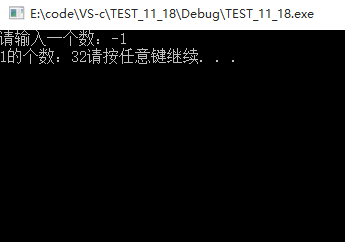```int main()
{
int i = 0;
int num = 0, count = 0;
printf("请输入一个数：");
scanf("%d", &num);
while
d62f
(num)
{
count++;
num = num&(num - 1);//一个数与他下一个数按位与，可以去掉最右边的1，此时一个二进制数中有几个1，就循环几次，最坏情况是循环32次。

}
printf("1的个数为：%d", count);
system("pause");

return 0;

}```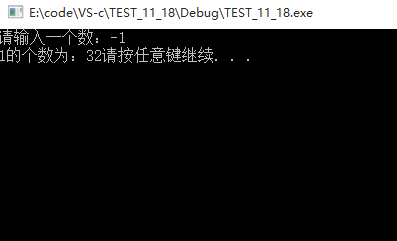```int main()
{
int a = { 0 };
int num = 0;
int i = 0;
printf("请输入一个数");
scanf("%d", &num);
for (i = 0; i < 32; i++)
{
a[i] = num & 1;
num = num >> 1;

}
printf("奇数序列");
for (i = 31; i >= 0; i -= 2)
{
printf("%d", a[i]);
}
printf("\n");
printf("偶数序列");
for (i = 30; i >= 0; i -= 2)
{
printf("%d", a[i]);
}
system("pause");
return 0;
}```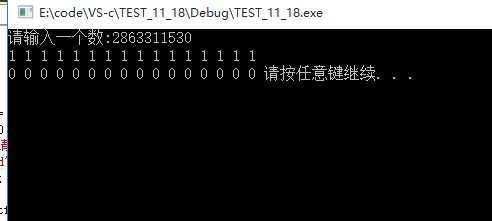```int main()
{
int num = 0;
int i = 0;
printf("请输入一个数:");
scanf("%d", &num);
for(i=31; i>0; i-=2)
{
printf("%d ", (num>>i)&1);
}
printf("\n");
for(i=30; i>=0; i-=2)
{
printf("%d ", (num>>i)&1);
}
system("pause");
return 0;
}```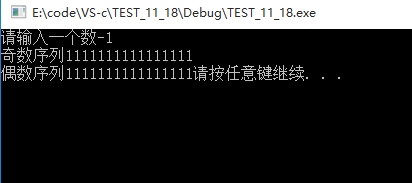```int main()
{
int num = 0;
int count = 0;
int i = 0, tmp = 0;
int a = { 0 };
printf("请输入一个数");
scanf("%d", &num);
tmp = num;
while (tmp)
{
count++;//整数的位数
tmp = tmp / 10;
}
for (i = 0; i < count; i++)
{
a[i] = num % 10;
num = num / 10;
}
for (i = count-1; i >= 0; i--)
{
printf("%d ",a[i]);
}
system("pause");
return 0;
}```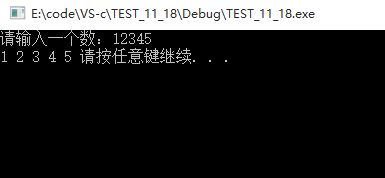```void Print(int n)
{
if (n > 9)
{
Print(n / 10);//递归打印每一个数，将每一个数拆开并打印
}
printf("%d ", n % 10);
}
int main()
{
int num = 0;
printf("请输入一个数：");
scanf("%d", &num);
Print(num);
system("pause");```

```int main()
{
int m = 0, n = 0;
int i = 0;
int count = 0;
printf("请输入两个数");
scanf("%d%d", &m, &n);
i = m^n;
while (i)
{
i=i&(i - 1);
count++;
}
printf("%d", count);
system("pause");
return 0;
}```